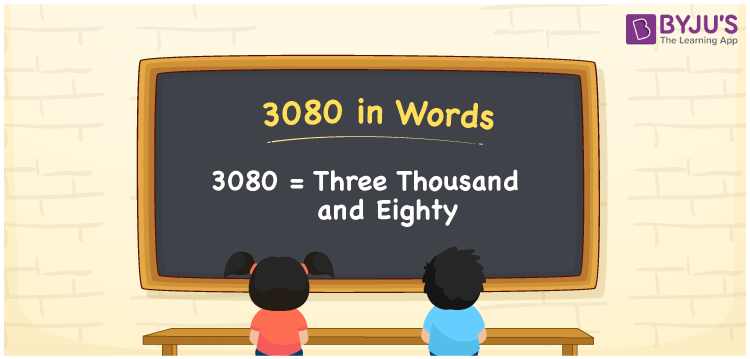# 3080 in Words

We can write 3080 in words as Three Thousand and Eighty. Suppose you bought a new keyboard that costs Rs. 3080, then you could say that “ I bought a new keyboard for Three Thousand and Eighty rupees”. In this article, you will learn how to convert the number 3080 into words in an exciting way. The number 3080 is used in expressions that relate to money, days, distance, length, weight and so on.

 3080 in words Three Thousand and Eighty Three Thousand and Eighty in Numbers 3080

## 3080 in English Words

We generally write numbers in words using the English alphabet. Thus, we can read 3080 in English as “Three Thousand and Eighty”.## How to Write 3080 in Words?

In this section, you will learn how to write the number 3080 in words. The given number 3080 has four digits, so we should make a place value chart that represents the place value for all these four digits. The order of place values of digits in a number is in accordance with the Indian numbering system, such as:

• Ones
• Tens
• Hundreds
• Thousands
• Ten-thousands
• Hundred-thousands or Lakhs

This can be done as follows.

 Thousands Hundreds Tens Ones 3 0 8 0

Thus, we can write the expanded form as:

3 × Thousand + 0 × Hundred + 8 × Ten + 0 × One

= 3 × 1000 + 0 × 100 + 8 × 10 + 0 × 1

= 3080

= One thousand five hundred

3080 is a natural number that precedes 3081 and succeeds 3079.

3080 in words – Three Thousand and Eighty

Is 3080 an odd number? – No

Is 3080 an even number? – Yes

Is 3080 a perfect square number? – No

Is 3080 a perfect cube number? – No

Is 3080 a prime number? – No

Is 3080 a composite number? – Yes

## Frequently Asked Questions on 3080 in Words

Q1

### How do you write 3080 in English?

We can write 3080 in English words as “Three Thousand and Eighty”.
Q2

### How do you write 3080 in words for a cheque?

For a cheque, we generally write 3080 in words as Three Thousand and Eighty rupees only.
Q3

### What number is 3080 in words?

The number 3080 is Three Thousand and Eighty in words.